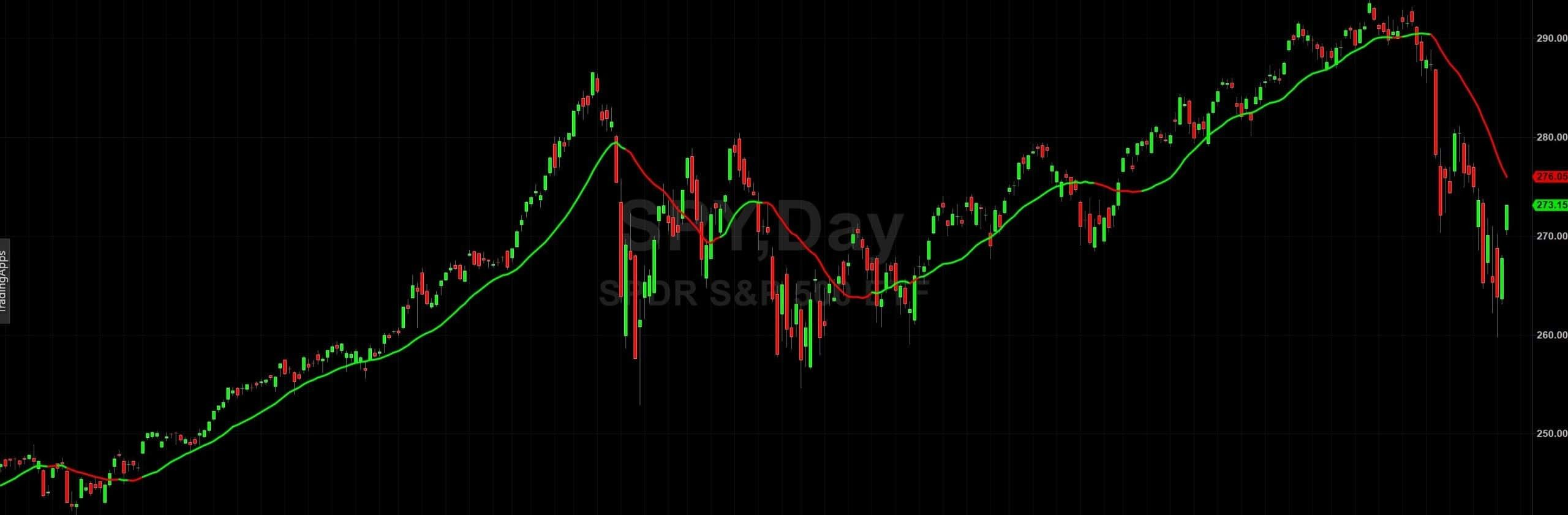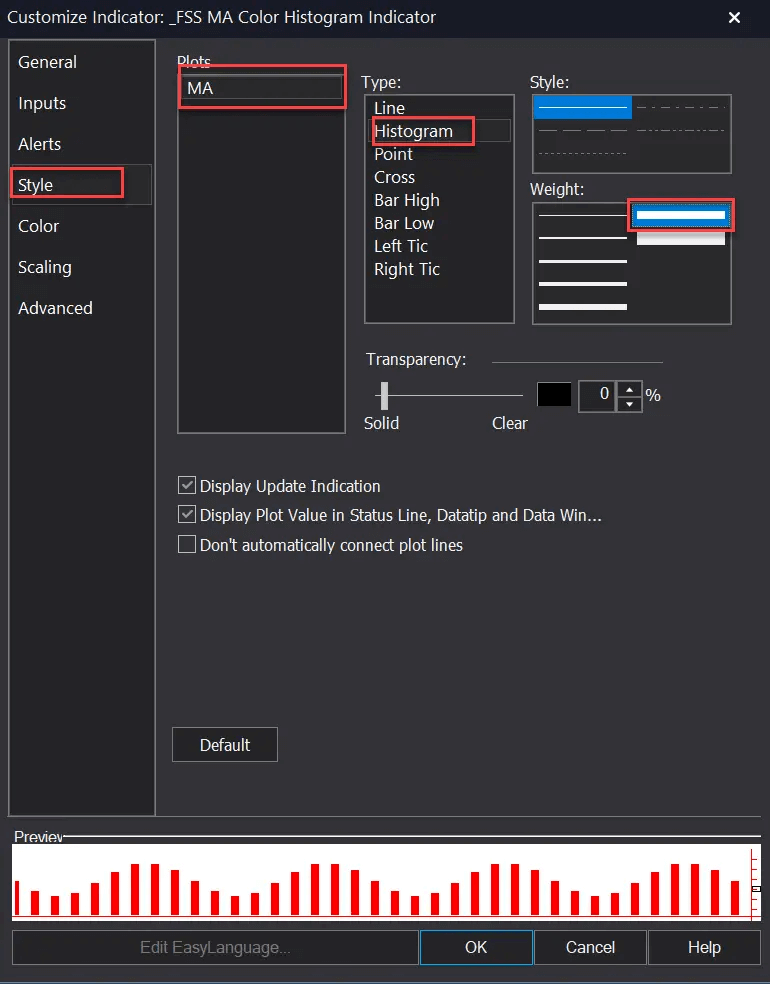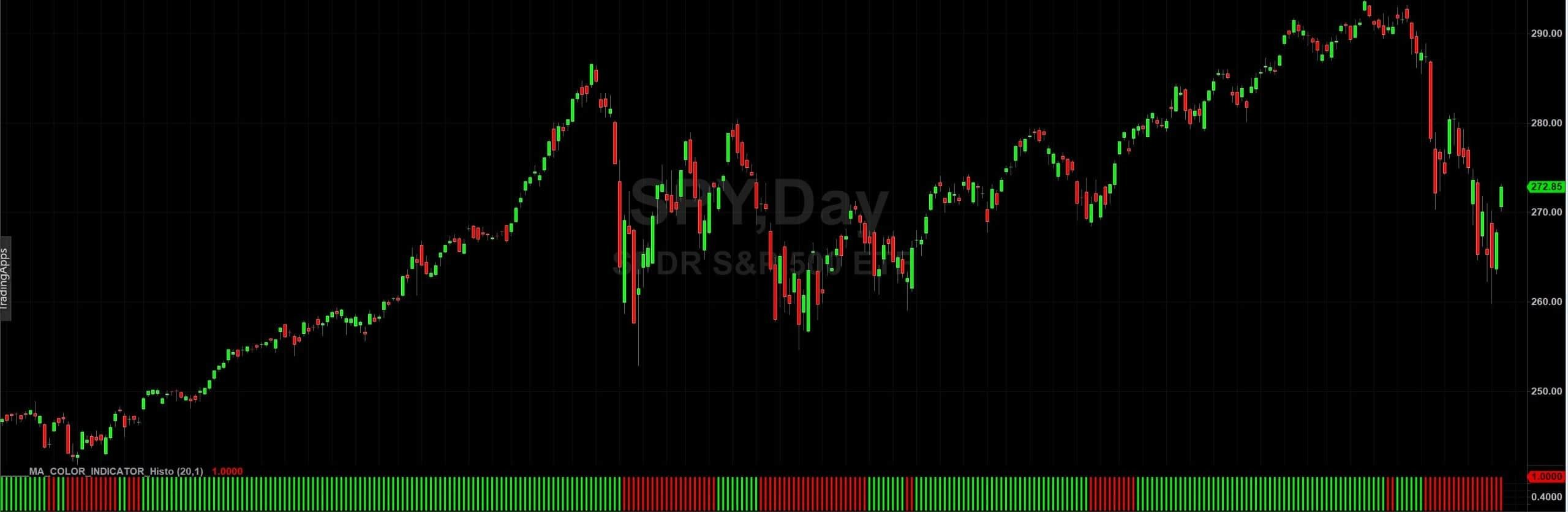# How to Transform any Indicator in Histogram

In this EasyLanguage Tutorial, we show you how to use the TradeStation Histogram visual mode. Many traders don't like to have the chart flooded with lines, drawings, and indicators.
They prefer to see the naked price. Let's take a simple example of a Moving Average from this link.
This moving average has an active slope function that indicates whether the direction is increasing or decreasing. Obviously, only this information can be viewed through a histogram. However, this information is very useful when we need a trend filter.Let's Start to transform a Moving Average into a TradeStation histogram.

First of all, we modify the Tradestation EasyLanguage code in this way:

``````Input:
MA_length(20);

vars:
MA(0),
color(white),
MA_histo(1);

MA=average(Close, MA_length);

If MA>MA and MA>MA then

color=Green;

If MA<MA and MA<MA then

color=Red;

Plot1( MA_histo, “MA”,color,0,3 );``````

The TradeStation Indicator continues to calculate the moving average but the command Plot1 instead of drawing the line with the value of the average price will draw the fixed amount "1".

This happens because with this indicator we are interested only in seeing the color that changes when the average is descending or ascending (red and green).

Some indicator settings must also be changed in Tradestation:Now you have only the TradeStation Histogram in your chart. The green-red histogram TradeStation can be used to display a large number of indicators.We can also create another histogram that shows us when prices are below or above the moving average.

Also in this case with a red and green histogram, we will have the red color when the prices will be below the moving average while the green color will be above.

``````Input:
MA_length(20),
MA_histo(1);

vars:
MA(0),
color(white);

MA = average(Close, MA_length);

If Close>MA
color=Green;

If Close<MA then
color=Red;

Plot1( MA_histo, “MA”,color,0,3 );``````

Do you want to learn EasyLanguage & PowerLanguage for TradeStation and MultiCharts?

Start here:

-- by Luca from blog financestrategysystem